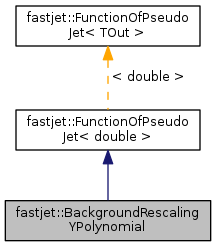FastJet  3.2.2
fastjet::BackgroundRescalingYPolynomial Class Reference

A background rescaling that is a simple polynomial in y. More...

#include <fastjet/tools/BackgroundEstimatorBase.hh>

Inheritance diagram for fastjet::BackgroundRescalingYPolynomial:[legend]
Collaboration diagram for fastjet::BackgroundRescalingYPolynomial:[legend]

Public Member Functions

BackgroundRescalingYPolynomial (double a0=1, double a1=0, double a2=0, double a3=0, double a4=0)
construct a background rescaling polynomial of the form a0 + a1*y + a2*y^2 + a3*y^3 + a4*y^4 More...

virtual double result (const PseudoJet &jet) const
return the rescaling factor associated with this jetPublic Member Functions inherited from fastjet::FunctionOfPseudoJet< double >
FunctionOfPseudoJet ()
default ctor

virtual ~FunctionOfPseudoJet ()
default dtor (virtual to allow safe polymorphism)

virtual std::string description () const
returns a description of the function (an empty string by default)

double operator() (const PseudoJet &pj) const
apply the function using the "traditional" () operator. More...

std::vector< double > operator() (const std::vector< PseudoJet > &pjs) const
apply the function on a vector of PseudoJet, returning a vector of the results. More...

Detailed Description

A background rescaling that is a simple polynomial in y.

Definition at line 219 of file BackgroundEstimatorBase.hh.

◆ BackgroundRescalingYPolynomial()

 fastjet::BackgroundRescalingYPolynomial::BackgroundRescalingYPolynomial ( double a0 = 1, double a1 = 0, double a2 = 0, double a3 = 0, double a4 = 0 )
inline

construct a background rescaling polynomial of the form a0 + a1*y + a2*y^2 + a3*y^3 + a4*y^4

The following values give a reasonable reproduction of the Pythia8 tune 4C background shape for pp collisions at sqrt(s)=7TeV:

• a0 = 1.157
• a1 = 0
• a2 = -0.0266
• a3 = 0
• a4 = 0.000048

Definition at line 234 of file BackgroundEstimatorBase.hh.

The documentation for this class was generated from the following files: